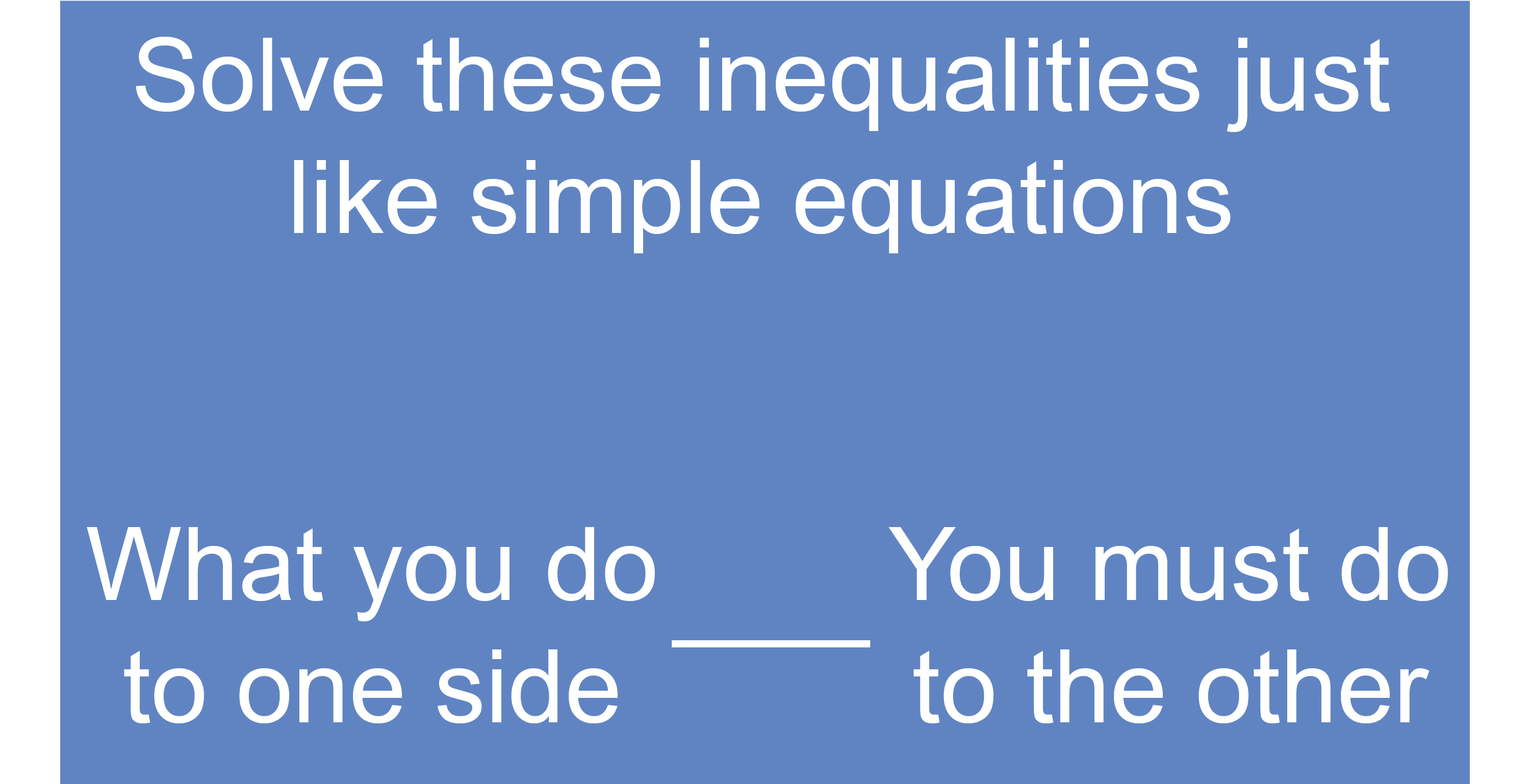# Solving less than or greater than expressions (two-part inequalities)

If there is a <,  >,  <= or >= in the equation then you:Examples

1.  Solve the inequality 4x-7<13

Add 7 to both sides to get x on its own.

4x-7+7<13+7

4x<20

Divide both sides by 4

(4x)/4<20/4

x<5

2.  Solve the inequality 7y\  – 34 ≤ 8

Add 34 to both sides to get y on its own.

7y-34+34<=8+34

7y<=42

Divide both sides by 7.

(7y)/7<=(42)/7

y<=6

3.  Solve the inequality 3(2y + 1) > 10

First multiply out the brackets and aim to get y on its own.

3xx2y+3xx1>10

6y+3>10

Subtract 3 from both sides.

6y+3\ –3>10-3

6y > 7

Divide both sides by 6

(6y)/6 > 7/6

y > 7/6

y > 1\1/6